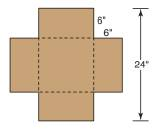Chapter 9.1, Problem 40E### Elementary Geometry for College St...

6th Edition
Daniel C. Alexander + 1 other
ISBN: 9781285195698

#### Solutions

Chapter
Section### Elementary Geometry for College St...

6th Edition
Daniel C. Alexander + 1 other
ISBN: 9781285195698
Textbook Problem
1 views

# As in Exercise 39, find the volume of the box if four congruent squares with sides of length 6 in. are cut from the corners of a rectangular piece of poster board that is 20 in. wide by 30 in. long.Kristine creates an open box by cutting congruent squares from the four corners of a square piece of cardboard that has a length of 24 in. per side. If the congruent squares that are removed have sides that measure 6 in. each, what is the volume of the box formed by folding and sealing the flaps?To determine

To find:

The volume of the box if four congruent squares with sides of length 6 in. are cut from the corners of a rectangular piece of poster board that is 20 in. wide by 30 in. long.

Explanation

Concept:

The volume of any solid is the region of space occupied by it and in general the volume of a solid is given by the product of its base area and altitude.

For a regular solid prism with base area B and altitude h, the volume is given by the formula

V=Bh.

Calculation:

Given,

Length of the square piece of the poster board =30 in

Width of the square piece of the poster board 20 in

The measure of the congruent squares that are removed from the four corners 6 in.

Since, the flaps are folder are folded the length of the flap (6 in.) become the height of the box formed.

Also, the length and width of the base of the box is given by subtracting the length of the square piece which are removed from the length and width of the poster board respectively.

Thus, the dimensions of the box is

l=30-6+6=30-12=18 in

### Still sussing out bartleby?

Check out a sample textbook solution.

See a sample solution

#### The Solution to Your Study Problems

Bartleby provides explanations to thousands of textbook problems written by our experts, many with advanced degrees!

Get Started

#### In problems 1-16, solve each equation. 20.

Mathematical Applications for the Management, Life, and Social Sciences

#### Simplify: 1218

Elementary Technical Mathematics

#### In Exercises 107-120, factor each expression completely. 112. x2 2x 15

Applied Calculus for the Managerial, Life, and Social Sciences: A Brief Approach

#### For y = x sin x, y = _____. a) x cos x b) x cos x + 1 c) cos x d) x cos x + sin x

Study Guide for Stewart's Single Variable Calculus: Early Transcendentals, 8th

#### Identify and describe the steps in the research process.

Research Methods for the Behavioral Sciences (MindTap Course List)